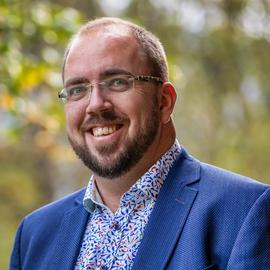# Biography

I am a professor of mathematics at UiT The Arctic University of Norway. I am currently also acting as Pro Dean for PhD education at the Faculty of Science and Technology. Currently, I have the pleasure to serve as Secretary of the SIAM Activity Group in Algebraic Geometry as well as member of the board of the Abel prize.

My research interests include real algebraic geometry, optimization, computational complexity, and computer algebra. I am leading the  Algebra group and I am also one of the initiators of the Lie-Størmer Center for Fundamental Structures in Computational and Pure Mathematics.

Interests
• Computational Complexity
• Real algebraic Geometry
• Symmetries in Computations
Education
• Habilitation in Mathematics, 2018

University of Konstanz

• Magister Artium (Philosophy), 2013

Goethe University Frankfurt

• PhD in Mathematics, 2011

Goethe University Frankfurt

• Dipl. Math. oec., 2006

Ulm University

• Master Mathematics, 2005

Université de Bordeaux

# ExperienceProfessor
UiT The Arctic University of Norway
Apr 2018 – Present Tromsø, NorwayVertretungsprofessur
University of Konstanz
Oct 2016 – Mar 2018 Konstanz, GermanyAalto Science Fellow
Aalto University
Oct 2012 – Feb 2016 Helsinki, FinlandPostdoctoral fellow
University of Konstanz
Apr 2011 – Sep 2012 Konstanz, GermanyResearch Assistant
Goethe University
Jan 2007 – Mar 2011 Frankfurt, Germany

# Publications

Quickly discover relevant content by filtering publications.
(2022). The poset of Specht ideals for hyperoctahedral groups. Arxiv.

(2022). Equivariant algebraic and semi-algebraic geometry of infinite affine space. arXiv:2203.11921.

(2022). Linear slices of hyperbolic polynomials and positivity of symmetric polynomial functions. arXiv:2203.08727.

(2022). Polynomial description for the T-Orbit Spaces of Multiplicative Actions. arxiv:2203.13152.

(2021). Symmetric ideals, Specht polynomials and solutions to symmetric systems of equations. Journal of Symbolic Computation.

(2021). Return of the plane evolute. arXiv:2110.11691.

(2021). # P-hardness proofs of matrix immanants evaluated on restricted matrices. arXiv preprint arXiv:2103.04934.

(2021). Symmetric nonnegative forms and sums of squares. Discrete & Computational Geometry.

(2021). Symmetry reduction in AM/GM-based optimization. SIAM J. Optimization.

(2021). Vandermonde varieties, mirrored spaces, and the cohomology of symmetric semi-algebraic sets. Foundations of Computational Mathematics.

(2020). Generalized eigenvalue methods for Gaussian quadrature rules. Annales Henri Lebesgue.

(2020). On the isotypic decomposition of cohomology modules of symmetric semi-algebraic sets: polynomial bounds on multiplicities. International Mathematics Research Notices.

(2020). Reflection groups and cones of sums of squares. arXiv preprint arXiv:2011.09997.

(2018). Reflection groups, reflection arrangements, and invariant real varieties. Proceedings of the AMS.

(2018). Real root finding for equivariant semi-algebraic systems. International Symposium on Symbolic and Algebraic Computation.

(2017). Bounding the equivariant Betti numbers of symmetric semi-algebraic sets. Advances in Mathematics.

(2017). Efficient algorithms for computing the Euler-Poincaré characteristic of symmetric semi-algebraic sets. Ordered Algebraic Structures and Related Topics, Contemporary Mathematics.

(2017). On irreducible components of real exponential hypersurfaces. Arnold Mathematical Journal.

(2016). Deciding positivity of multisymmetric polynomials. Journal of Symbolic Computation.

(2016). Symmetric semi-algebraic sets and non-negativity of symmetric polynomials. Journal of Pure and Applied Algebra.

(2013). Exploiting symmetries in SDP-relaxations for polynomial optimization. Mathematics of Operations Research.

(2012). A note on extrema of linear combinations of elementary symmetric functions. Linear and Multilinear Algebra.

(2012). On the degree and half-degree principle for symmetric polynomials. Journal of Pure and Applied Algebra.

(2008). Positive Polynome und semidefinite Optimierung. Jahresbericht der DMV.

(2006). On extreme forms in dimension 8. Journal de théorie des nombres de Bordeaux.

# Contact

• cordian.riener@uit.no
• +47 77 64 40 90
• Forskningsparken, Tromsø, 9037
• Forskningsparken 1 4th floor B425
• DM Me
• Skype Me